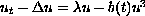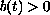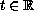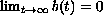Electron. J. Diff. Eqns., Vol. 2002(2002), No. 72, pp. 1-20.

### Pullback permanence for non-autonomous partial differential equations Jose A. Langa & Antonio Suarez

Abstract:
A system of differential equations is permanent if there exists a fixed bounded set of positive states strictly bounded away from zero to which, from a time on, any positive initial data enter and remain. However, this fact does not happen for a differential equation with general non-autonomous terms. In this work we introduce the concept of pullback permanence, defined as the existence of a time dependent set of positive states to which all solutions enter and remain for suitable initial time. We show this behaviour in the non-autonomous logistic equation, withfor all,. Moreover, a bifurcation scenario for the asymptotic behaviour of the equation is described in a neighbourhood of the first eigenvalue of the Laplacian. We claim that pullback permanence can be a suitable tool for the study of the asymptotic dynamics for general non-autonomous partial differential equations.

Submitted May 14, 2001. Published August 8, 2002.
Math Subject Classifications: 35B05, 35B22, 35B41, 37L05.
Key Words: Non-autonomous differential equations, pullback attractors, comparison techniques, permanence.

Show me the PDF file (303K), TEX file, and other files for this article.Jose A. Langa Departamento de Ecuaciones Diferenciales y Analisis Numerico, Universidad de Sevilla, Apdo. de Correos 1160, 41080-Sevilla, Spain e-mail: langa@numer.us.es Antonio Suarez Departamento de Ecuaciones Diferenciales y Analisis Numerico, Universidad de Sevilla, Apdo. de Correos 1160, 41080-Sevilla, Spain e-mail: suarez@numer.us.es